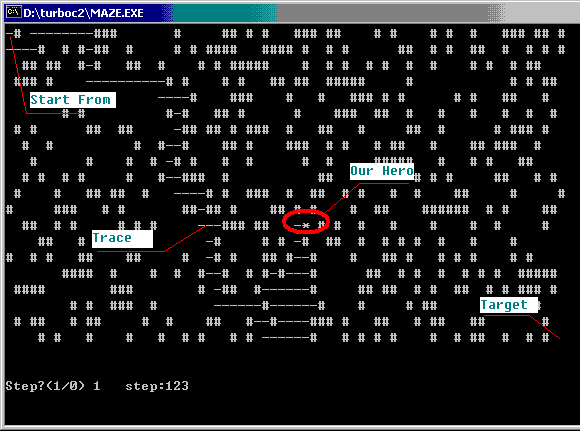A website for better software and better life in Internet!MY WEBChinese URLsGreeting CreatorInput Tips EverydaySource CodeJava Applet GamesVisit webpage in VCM3U Music List EditorInput Tips everydayCombox in HTMLSelf-made Screen SaverModel of Neural NetworkDisplay Chinese CharactersMouse in Maze, a classic game in mathSerial Port Communication

Source Code -> Mouse in Maze

## Mouse in Maze

That's a classic Mathematical program, asking you to help a mouse to find its way through the arbitrary maze.
It's feasible to find a best route using the method of Back Propogation Algorithm of Neural Network. But here I use a particular method to work it out. The philosophy behind the solution is the fact that the mouse don't know anything about the maze. So it's like a robot: test every direction facing the mouse. How to do that?step 1, if to the left side of the mouse,it is a wall? If yes, go to the left space, and repeat step 1.
step 2, if the mouse can go to the left? if yes, go to the below space and repeat step 1.
.
.
step n, if the mouse can not go out, return to the space where it came from, and try again.

Source code is not too long, but it really works and it took me long time to figue it out! So I share it here, hope you can enjoy it.
Comment:
In the first part of the source code you can find the path in the maze is randomized, and I put 9 spaces near the start point and 9 spaces near the end point to help the mouse.
You can use "STEP" by input "1" at the beginning of the software so that the mouse will stop after every step and wait for you to click any key. By this mean you can clearly see how the mouse steps into the unknown future, or return to the last space to find a better way. It's very interesting to observe this intelligence lovely mouse.
You can not compile this application in Visual C, because some functions use to show the step, such as gotoxy(), is not allowed in Visual C. So I made maze.exe using Turbo C, you can download it directly.
Here is the source code:
```#include < stdlib.h>
#include < time.h>
#include < stdio.h>
#include < conio.h>
main()
{int x,y,a;
char o;
int c,d,four,k=0,i,end,step;
randomize();
/* printf("Do you want it run or step?(0/1)");
scanf("%d",&step);*/
clrscr();
for(x=0;x < 72;x++){
for (y=0;y <  22;y++){
if ((y==0)||(x==0)||(x==71)||(y==21)) {
a[x][y]=11;
continue;}
if (random(3)==0) {
a[x][y]=11;
gotoxy(x,y);
printf("#");
}
else
a[x][y]=1;
}
}

for(y=1;y <  10;y++)
{ a[y]=1;
gotoxy(1,y);
printf(" ");
a[21-y]=1;
gotoxy(70,21-y);
printf(" ");
}
x=1;
y=1;
end=0;
gotoxy(1, 23);
printf("Step?(1/0) ");scanf("%d", &step);
//Above is to prepare the Maze. Now, our little mouse is comming.

while((x < 70)||(y < 20)) {

c=x;d=y;four=0;
do{
k++;
if ((a[x+1][y]==1)||((a[x+1][y]%7==0)&&(a[x][y]%2!=0)&&(four==1))) {
a[x][y]=a[x][y]*2;
x=x+1;
break;
}
if ((a[x][y+1]==1)||((a[x][y+1]%5==0)&&(a[x][y]%3!=0)&&(four==1))) {
a[x][y]=a[x][y]*3;
y=y+1;
break;
}
if ((a[x][y-1]==1)||((a[x][y-1]%3==0)&&(a[x][y]%5!=0)&&(four==1))) {
a[x][y]=a[x][y]*5;
y=y-1;
break;}
if ((a[x-1][y]==1)||((a[x-1][y]%2==0)&&(a[x][y]%7!=0)&&(four==1)))
{a[x][y]=a[x][y]*7;
x=x-1;
break;
}
four++;
if (four == 2){
gotoxy(25, 23); printf("Cannot go out!");
end=1;
break;}

if (k>3000) {
gotoxy(30, 23); printf("Too long!");
end=1;
break;}
}while(1);
if (end==1) break;
/* gotoxy(x,y);printf("*");*/
gotoxy(c,d);printf("-");
gotoxy(16, 23); printf("step:%d ", k);
gotoxy(x,y); printf("*");
if (step==1) getch();
}/* no end point*/
if (end==1) printf("Error.");
else printf("ok!");
getch();
}/*end main()*/

```
Are you understand the program? I use Prime number to record every space the mouse passed. If there's a space (value==1) near it, go. If it has check 4 directions but can not find a space, then four==1, the mouse must go the the place where it go from. After it returned to the last place, it will look for space (value==1) again, or go to the last palce again....
Do you like this strategy? If you have a new strategy for the mouse, realize it.Contact InfoUser SupportSales QuestionWebmaster